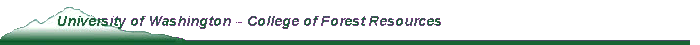Abstract

Equivalence of the expectation and cost compounding methods of forest valuation - Faustmann revisited

Bruce Manley and B. Bruce Bare

In 1849, Faustmann showed that the value of a forest stand could be calculated either by compounding costs or discounting future cashflows. The linkage between the two approaches was the inclusion in both of the cost of land based on Land Expectation Value (LEV). In this paper a forest investment example is used to examine the comparability of compounding costs and discounting future cashflows to estimate Crop Expectation Value (CEV) in the context of the NZIF Forest Valuation Standards.

When valuation is based on pre-tax cashflows, the cost compounding method will give the same value as the CEV estimated by discounting cashflows provided the same cost and revenue assumptions are used to estimate both. This is not the case when the current crop has sub-optimal management or when the cost compounding method includes non-recurring costs that only occur in the first rotation. In these cases, the cost compounding method overestimates CEV.

When valuation is based on post-tax cashflows, the cost compounding method will only provide the correct CEV if the present value of the tax deduction associated with the purchase price (i.e., cost of bush or cost of timber deduction) is added to the sum of compounded costs.

To Return to:Prof Bare's Page, College of Forest Resources